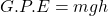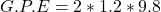## Someone please help me! And I will mark you as brainlist!! 1) A ball with a mass of 2.0 kg is thrown upward 1.2m calculate its g

Question

1) A ball with a mass of 2.0 kg is thrown upward 1.2m calculate its gravitational potential energy into two significant figures. Gravity = 9.8 N/kg

• 2.4 J
• 1.4 J
• 120 J
• 24 J

2) Translation kinetic energy is defined as the energy of a system due to the motion of the system’s…

• center of mass
• axis of symmetry
• molecules
• rotational movement

in progress 0
5 months 2021-08-26T01:29:28+00:00 1 Answers 8 views 0

1. G.P.E = 24 J

2. center of mass

Explanation:

Given the following data;

Mass = 2kg

Height, h = 1.2m

Acceleration due to gravity = 9.8 N/kg or m/s².

To find the gravitational potential energy;

Gravitational potential energy (GPE) is an energy possessed by an object or body due to its position above the earth.

Mathematically, gravitational potential energy is given by the formula;Where;

• G.P.E represents potential energy measured in Joules.
• m represents the mass of an object.
• g represents acceleration due to gravity measured in meters per seconds square.
• h represents the height measured in meters.

Substituting into the formula, we have;G.P.E = 23.52 to 2 S.F = 24 Joules.

Translation kinetic energy is defined as the energy of a system due to the motion of the system’s center of mass. The center of mass is typically where the mass of the object or particle is concentrated.